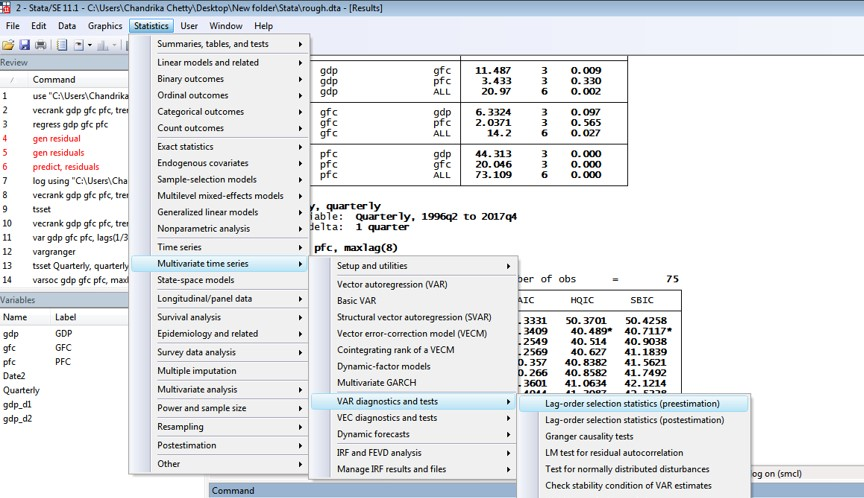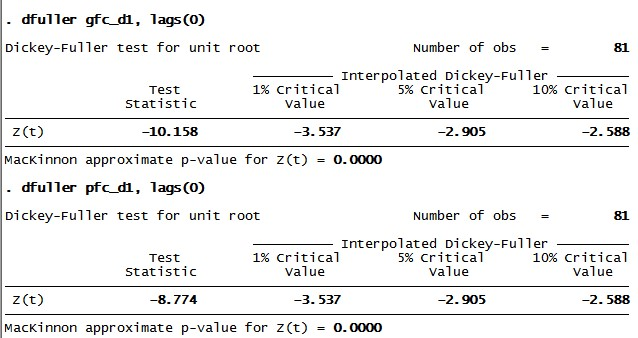# Lag selection and stationarity in VAR with three variables in STATA

The previous article explained how to perform the lag selection, Johansen co-integration test and Vector Auto Regression (VAR) with two variables, Gross Domestic Product (GDP) and Private Final Consumption (PFC). This article incorporates Gross Fixed Capital Formation (GFC) and again performs the lag selection test and check for stationarity for both, GFC and PFC. Thus this article incorporates the VAR with three variables in STATA. The process also includes Johansen cointegration test for the model including all three-time series.

While running the regression for time series data, it is important to include the lagged values of the dependent as well as independent variables. For instance, last year’s GDP may be correlated to this year’s GDP, this showing lagged values. Thus if past values affect today’s values then more lags will be necessary. Therefore, to determine the exact level of lags, the first step is to perform lag selection criteria in STATA.

## Lag selection

1. Click on ‘Statistics’ on Result window
2. Choose ‘Multi-variate Time Series’
3. Click on ‘VAR Diagnostic and Test’
4. Select ‘Lag-order selection statistics’.Figure 1: Lag selection criteria for VAR with three variables in STATA

After selecting the lag-order selection statistics, a ‘varsoc’ window will open in STATA (figure below). Select two components on the main windo; the list of dependent variables (GDP, GFC and PFC), and the maximum lag order.Figure 2: Varsoc window in STATA for lag selection for VAR with three variables

Select the maximum number of lags to check; for instance, “8”. Further in ‘Dependent variables’ option, select three main variables GDP, GFC and PFC.Figure 3: Varsoc window in STATA for lag selection for VAR with three variables

The results will appear in the output window (figure below). The STATA command for lag selection is also visible here. Alternatively, use the below command to generate the result:

`varsoc gdp gfc pfc, max lag (8)`Figure 4: Lag selection results in STATA for VAR with three variables

In the result table, the number of lags in the first column and the parameters for optimal lags like Final Prediction Error (FPE), Akaike information criterion (AIC), Hannan Quinn Information Criteria (HQIC) and Schwartz Information Criteria (SBIC) are visible. STATA with this command computes four information criteria as well as a sequence of likelihood ratio (LR) tests.

## Identify the number of lags

To identify the number of lags, select the values showing “”. For instance, FPE shows value at lag 8 and carries the sign “”. Therefore, the lag as per FPE criteria is 8. In order to select the parameter with optimal lags for VAR, follow the majority number. That means, if three out of four parameters show the same number of lags, let’s say 8, then take 8 lags. However, in this case, it is not possible to follow the majority since two parameters show 1 and the other two show 8. Therefore, follow the AIC and FPE criteria, since the number of observations is more than 60.

## Stationarity

This case follows the same steps for GFC and PFC used in the case of ARIMA where stationarity of GDP time series was checked. The figure below shows the time series graph of PFC and GFC.Figure 5: Time series plot for GFC and PFC

As the graph shows, trends for both GFC and PFC is upward, indicating that mean and variances of both variables are non-constant. That means both the series are non-stationary. For more clarity, use the augmented Dickey-Fuller test. In order to transform the times series PFC and GFC into stationary, apply differencing. Following the below two commands:

1. Generate gfc_d1 = d1.gfc
2. Generate pfc_d1 = d1.pfc

The first differencing series of GFC (gfc_d1) and PFC (pfc_d1) is generated. To ascertain the stationarity, again perform augmented Dickey-Fuller test. The figure below shows the result for GFC (gfc_d1) and PFC (pfc_d1):Figure 6: Augmented Dickey Fuller test for PFC and GFC

The p values for both differenced series of GFC (gfc_d1) and PFC (pfc_d1) is close to zero, which means the null hypothesis of non-stationarity can be rejected and first differenced time series are stationary.

This article shows how to perform lag selection and stationarity test in a VAR with three variables GDP, PFC and GFC. The next article shows the co-integration test to choose the correct type of VAR model.

### Divya Dhuria

Research analyst at Project Guru
DIvya has a keen interest in policy making and wealth management. She is a Master in Economics from Gokhale Institute of Politics and Economics. She has been trained in the econometric techniques to assess different possible economic relationships. She hascontributed to the working paper on National Rural Health Mission at Institute of economic growth, Delhi. Along with academical growth, she likes to explore and visit different places in her spare time.

#### Latest posts by Divya Dhuria (see all)We are looking for candidates who have completed their master's degree or Ph.D. Click here to know more about our vacancies.